Refer to our Texas Go Math Grade 4 Answer Key Pdf to score good marks in the exams. Test yourself by practicing the problems from Texas Go Math Grade 4 Lesson 16.3 Answer Key Add and Subtract Money.

Essential Question

How can you use addition and subtraction in problems involving money?
You can add and subtract decimals the same way you add and subtract whole numbers if you line up the decimal points first.

Unlock the problem

The balance in Marsha’s savings account is $423.54. She makes a deposit of$58.95. What is the balance in her savings account now?

The amount of money that is in an account after a deposit or withdrawal is made is called the balance. A deposit is when money is added to the balance of an account. A withdrawal is when money is subtracted from the balance of an account.

Math Idea: You can add and subtract decimals the same way you add and subtract whole numbers if you line up the decimal points first.

Use addition to find the balance after a deposit.

STEP 1
Line up the decimal points.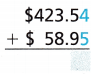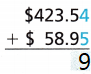STEP 2
Add the tenths. Regroup as needed.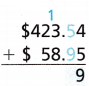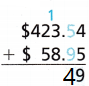STEP 3
Add the ones, tens, and hundreds. Place the decimal point in the sum.So after the deposit, the balance in Marsha’s savings account is __________.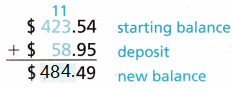So after the deposit, the balance in Marsha’s savings account is $484.49 Use subtraction to find the balance after a withdrawal. Two weeks later, Marsha withdraws$75.34. What is the balance now?

STEP 1
Subtract the hundredths.STEP 2
Subtract the tenths.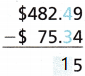STEP 3
Subtract the ones, tens, and hundreds. Place the decimal point in the difference.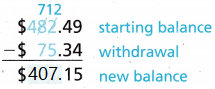So after the withdrawal, the balance in Marsha’s savings account is _____________ .
So after the withdrawal, the balance in Marsha’s savings account is $407.15 Share and Show Question 1. Mrs. Freeman has a checking account with a balance of$442.37. She writes a check for $63,92. Then she makes a deposit of$350.00. What is the final balance in her checking account after writing the check and making the deposit?

First use subtraction to find the balance after writing the check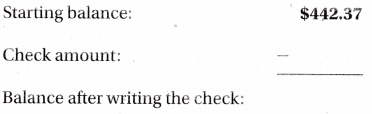Then use addition to find the final balance after making the deposit,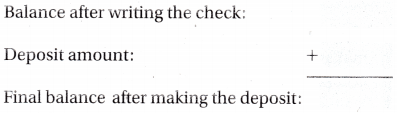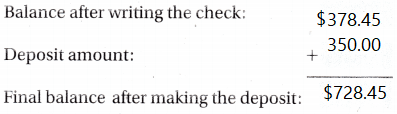So, Mrs. Freeman’s final balance is ___________ .
So, Mrs. Freeman’s final balance is $728.45 Question 2. Jeremy has a school store account with a balance of$16.82. He spends $2.75 to buy a new marker. What is the balance of Jeremy’s school store account? Answer: 14.07 is the balance Explanation: 16.82 – 2.75 = 14.07 14.07 is the balance of Jeremy’s school store account Question 3. Sarah has$789.59 in her checking account. She makes a deposit of $65.32. Then she writes a check for$105.00. What is the balance of her checking account after she writes the check?
$619.27 is the balance of her checking account after she writes the check Explanation: Sarah has$789.59 in her checking account. She makes a deposit of $65.32. 789.59 – 65.32 = 724.27 Then she writes a check for$105.00.
724.27 – $105.00. =$619.27

Problem Solving

Question 4.
H.O.T. Analyze Lana’s checking account had a balance of $589.33 at the beginning of the month. She made no deposits that month and the bank charged no fees. At the end of the month, her balance was$472.58. How much had she written in checks that month? Explain.
$116.75 she written in checks that month. Explanation:$589.33 – $472.58 =$116.75

Question 5.
Multi-Step Ponce went shopping with $20. He bought a shirt for$7.89 at one store and a DVD for $11.79 at another. How much money did he have left after he bought the shirt and the DVD? Answer: 0.32$ money that he have left after he bought the shirt and the DVD
Explanation:
7.89 + 11.79 = 19.68
$20 – 19.68 = 0.32 Problem Solving Question 6.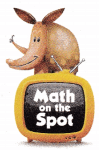H.O.T. Multi-Step Mitch began the month with$733.12 in his savings account. He deposited his earnings of $24.00 for babysitting and$31.00 for doing chores. That same month, he withdrew $331.76. How much money was in his account at the end of the month? Answer:$436.36 money was in his account at the end of the month
Explanation:
$24.00 + 31.00 = 35 733.12 + 35 = 768.12$768.12 – 331.76 = $436.36 Question 7. H.O.T. Multi-Step Max has$720.67. He spends $435.22 on an HD television and$102.65 on a DVD player. How much money does Max have now?
$182.8 money that Max have now Explanation: 435.22 + 102.65 = 537.87$720.67 – 537.87 = $182.8 Question 8. H.O.T. Multi-Step Paul wants to know what his balance was at the beginning of the month. He knows his balance was$978.45 at the end of the month. During the month, he withdrew $332.70 and deposited$500.00. What was his beginning balance? Explain how you got your answer.
$1145.75 was his beginning balance Explanation:$978.45 – $332.70 = 645.75 645.75 + 500.00 =$1145.75

Question 9.
H.O.T. Multi-Step Nick wants to start a lawn mowing business. He will pay his workers $15.25 to mow a lawn. It costs$4.77 for gas to mow one lawn. If Nick charges his customer $32.00 to mow the lawn, how much will he have left after paying his worker and paying for the gas? Answer: 21.52 he have left after paying his worker and paying for the gas. Explanation: 15.25 – 4.77 = 10.48$32.00 – 10.48 = 21.52

Question 10.
Multi-Step At the end of March, Vicki had an account balance of $185.78. Since then, she has written two checks, one for$25.50 and another for $18.34. How much is in her account now? (A)$160.28
(B) $141.94 (C)$178.62
(D) $167.44 Answer:$141.94 is in her account now
Explanation:
$25.50 + 18.34 = 43.84 185.78 – 43.84 = 141.94 Question 11. Mrs. Jackson bought lunch for herself and two friends. The lunches cost$8.25, $6.00, and$10.50. How much do the three lunches cost all together?
(A) $24.00 (B)$11.25
(C) $38.25 (D)$24.75
Explanation:
8.25 + 6.00 = 14.25
14.25 + 10.50 = 24.75

Question 12.
Multi-Step Doug bought a pair of sneakers for $47.82 and a shirt for$11.36. If Doug had $100 before his purchase, how much money does he have left now? (A)$38.18
(B) $59.18 (C)$40.82
(D) $35.82 Answer: C Explanation: 47.82 + 11.36 = 59.18 100 – 59.18 = 40.82 TEXAS Test Prep Question 13. Mrs. Proctor worked for a company that gave her$300 for travel. She spent $114.59 for a hotel room,$15.97 for lunch and $25.83 for dinner. How much money did she have left at the end of her trip? (A)$227.21
(B) $156.39 (C)$143.61
(D) $372.79 Answer: C Explanation: 114.59 + 15.97 = 130.56 130.56 + 25.83 = 156.39 300 – 156.39 =$143.61

### Texas Go Math Grade 4 Lesson 16.3 Homework and Practice Answer Key

Question 1.
Kim had $472.15 in her checking account. She deposited$188.50 and wrote a check for $263.89. How much is in Kim’s checking account now? Answer:$396.76 is in Kim’s checking account now.
Explanation:
472.15 + 188.50 = 660.65
660.65 – 263.89 = 396.76

Question 2.
Julia sold her bicycle for $88.50. She sold her bicycle helmet for$8.75. She bought a basketball for $35.82. How much money does Julia have now? Answer:$61.43 money that Julia have now
Explanation:
88.50 + 8.75 = 97.25
97.25 – 35.82 = 61.43

Question 3.
Nick’s lunch cost $14.75. Tax was$1.03 and he left a tip of $2.50. How much did Nick pay for his lunch? Answer:$18.28 Nick pay for his lunch
Explanation:
14.75 + 1.03 = 15.78
15.78 + 2.50 = 18.28

Question 4.
Mario buys a shirt for $28.95 and a pair of socks for$4.29. He gives the clerk at the store two $20 bills. How much change should Mario receive? Answer: Explanation: Problem Solving Question 5. Susan bought a hat and gave the salesperson at the store$50.00. She got $13.72 back in change. How much did Susan pay for the hat? Explain how you got your answer. Answer: 36.28 Susan pay for the hat. Explanation: 50.00 – 13.72 = 36.28 Question 6. At the beginning of the month, Javier had$671.90 in his checking account. At the end of the month, he had $422.15. He knows he wrote 2 checks. One check was for$118.42. How much was the other check for? Explain how you got your answer.
131.33 was the other check
Explanation:
118.42 + 422.15 = 540.57
671.90 – 540.57 = 131.33

Lesson Check

Question 7.
Brad had $515.97 in his savings account at the beginning of the month. At the end of the month he had$648.47 in the account. If Brad withdrew no money that month, how much money did he deposit into his savings account that month?
(A) $133.50 (B)$1,164.44
(C) $1,153.34 (D)$132.50
Explanation:
648.47 – 515.97 = 132.50
132.5 money that he deposit into his savings account that month

Question 8.
Leslie bought a magazine for $5.98. She paid with a$10.00 bill. How much change did Leslie receive?
(A) $5.02 (B)$4.12
(C) $4.02 (D)$5.12
Explanation:
10.00 – 5.98 = 4.02

Question 9.
Multi-Step Carina had $283.20 in her checking account. She wrote checks for$28.50 and $35.67. How much is left in Carina’s checking account? (A)$219.03
(B) $262.13 (C)$230.03
(D) $219.13 Answer: A Explanation: 28.50 + 35.67 = 64.17 283.20 – 64.17 = 219.03 left in Carina’s checking account Question 10. Multi-Step Allen wants to buy a television for$789.98. He has $512.16 in savings. He can save another$123.62 this week. How much more money does he need to buy the television?
(A) $143.20 (B)$401.44
(C) $154.20 (D)$635.78
Explanation:
512.16 + 123.62 = 635.78
789.98 – 635.78 = 154.2

Question 11.
Multi-Step Lucy spent $16.08 for a dog collar and$43.57 for a dog bed. She has $25.45 left. How much money did Lucy have before she went shopping? (A)$85.10
(B) $7.06 (C)$74.90
(D) $75.12 Answer: A Explanation: 16.08 + 43.57 = 59.65 59.65 + 25.45 = 85.1 Lucy have before she went shopping Question 12. Multi-Step Barry had$68.17 in his pocket. His friend Jack returned $20.00 he borrowed from Barry. Then Barry spent$32.91 on groceries. How much money does Barry have now?
(A) $17.26 (B)$55.26
(C) $56.26 (D)$56.06